Search

Systems of Linear Equations: Examples (page 7 of 7)

Sections: Definitions, Solving by graphing, Substitition, Elimination/addition, Gaussian elimination.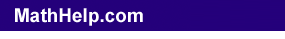While math-class systems usually have integer solutions, sometimes (especially for word problems) you'll see solutions involving fractions.

• Solve the following system of equations using Gaussian elimination:
• 2x + y + 3z = 1
2
x + 6y + 8z = 3
6
x + 8y + 18z = 5

I think I'll use the first row to clear out the x-terms from the second and third rows: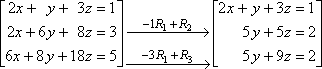Technically, I should now divide the first row by 2 to get a leading 1, but that will give me fractions, and I'd like to avoid that for as long as possible. Instead, I'll move on to using the second row to clear out the y-term from the third row: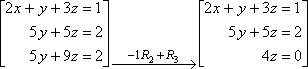I can divide the third row by 4: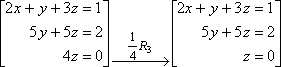To be technically correct, I'll now divide the second row by 5 and the first row by 2: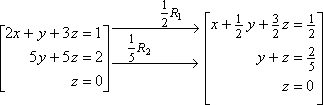(You might want to check with your instructor regarding how particular he's going to be about proper form. Do you "have" to show all 1's for the leading coefficients, or it is acceptable to avoid fractions?)

Back-solving, I get:

y + (0) = 2/5
y = 2/5

x + ( 1/2 )( 2/5 ) + ( 3/2 )(0) = 1/2
x + 1/5 = 1/2
x = 3/10

Then the solution is (x, y, z) = ( 3/10, 2/5, 0).

Warning: While I didn't show my scratch work on this last problem, I did have to do the scratch work. Please use scratch paper and write things out; don't try to do this stuff in your head; there are just way too many opportunities for errors. (Ya wanna know how many mistakes I made while writing this lesson? Don't even get me started!)

Here's another example:

• Solve the following system:
• 3x +    y  6z = 10
2
x +    y  5z =  8
6
x  3y + 3z =    0

I think I'll use the second row to work on the x-terms in the first and third rows. I'll be able to clear out the third row, and I'll be able to produce a 1x as the leading term in the first row. This will let me finish the job of clearing out the x-column, and I'll be able to do it without having to deal with fractions: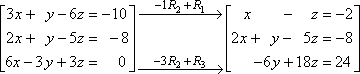(Many instructors would teach you always to divide through on one of the rows to get a leading coefficient of 1, but I would rather take an extra step or two and use addition to get a leading 1. That's just a personal preference, but I'm sure you can see the advantage of avoiding fractions for as long as possible. It can really cut down on computational errors.)

Now I'll use that nice leading x in the first row to clear out the leading term in the second row. While I'm at it, I'll also divide the third row by 6, to get smaller numbers: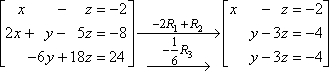Hm... The second and third rows are the same. I can use the second row to clear out the third row entirely: Copyright © Elizabeth Stapel 2003-2011 All Rights Reserved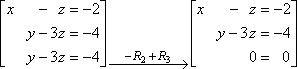Thinking back to the two-variable case, getting a line like "0 = 0" (which is true, but unhelpful) means that this is a dependent system, and the solution is going to have variables in it. If you get into linear algebra much, you will learn that the answer above means that the solution is a line in three-dimensional space rather than a single point. For now, all you need to know is how to write the solution. To find the solution, I have to solve the two remaining equations for x and y in terms of z:

x  z = 2
x = z  2

y  3z = 4
y = 3z  4

(x, y, z) = (t  2, 3t  4, t)

Remember that your book may use some variable other than "t", and that t is just standing in for z. This form of the solution just says that z is whatever value you chose, and then x is two less than that and y is four less than three times as much as z.

Content Continues Below

• Solve the following system of equations:
• x      + z = 1
x + y + z = 2
x  y + z = 1

You should be getting the hang of things by now, so I'll just show the steps that I used: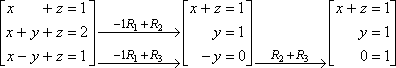As soon as I get a nonsense row (like "0 = 1"), I know that this is an inconsistent system, and I can quit.

inconsistent system: no solution

Remember the difference between the two special cases: A trivial row (such as "0 = 0") means you have a dependent system with a solution that contains variables; a nonsensical row (such as "0 = 1") means you have an inconsistent system with no solution whatsoever. Don't confuse these; they are (Warning!) common trick questions on tests.

Depending on the course, you might now move on to using matrices for solving systems of equations. If you do, the techniques you'll be learning for matrices will likely be very similar to what you have seen in this lesson.

<< Previous  Top  |  1 | 2 | 3 | 4 | 5 | 6 | 7  |  Return to Index

 Cite this article as: Stapel, Elizabeth. "Systems of Linear Equations: Examples." Purplemath. Available from     https://www.purplemath.com/modules/systlin7.htm. Accessed [Date] [Month] 2016